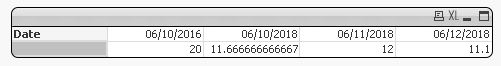# App Development

Announcements
April 22, 2PM EST: Learn about GeoOperations in Qlik Sense SaaS READ MORE
cancel
Showing results for
Did you mean:Contributor III

## Accumulated average and set expression

Hi, folks.

I need to implement a kind of "accumulated"/moving average. I am stuck with the set expression to determine the calculation universe I will use.

I have some ticket data as the example below:

RecNoSnapshot_DateTicketTicket_Age
106/10/2018A10
206/10/2018B5
36/10/2016C20
406/11/2018A10
506/11/2018B6
606/11/2018C21
706/12/2018A10
806/12/2018B7
906/12/2018C21
1006/12/2018D1

The user must be able to select whatever Snapshot_Date she wants. Qlik should calculate the "accumulated average age" up to the selected Snapshot_Date. Some examples:

User Snapshot_Date selection: 06/10/2018

Result: 11.67

Calculation: (10 + 5 + 20) / 3

Corresponding RecNo: 1 to 3

User Snapshot_Date selection: 06/11/2018

Result: 12

Calculation: (10 + 5 + 20 + 10 + 6 + 21) / 6

Corresponding RecNo: 1 to 6

User Snapshot_Date selection: 06/11/2018 and 06/12/2018

Result: 10.86

Calculation: (10 + 6 + 21 + 10 + 7 + 21 + 1) / 7

Corresponding RecNo: 4 to 10

I try using something like the calculation below, to no avail.

```Sum({\$ <Snapshot_Date = {"<=\$(=Max([Snapshot_Date]))"}>} Ticket_Age) / Count({\$<snapshot_date = {"<=\$(=Max(Snapshot_Date))"}>} Ticket)
```

1 Solution

Accepted SolutionsCreator III

Hi Al,

I used the following expression.

Rangesum(sum({\$<Date={"<=\$(max(num(Date)))"}>} Value),before(sum({<Date={"<=\$(max(num(Date)))"}>} Value),1,ColumnNo()))

/Rangesum(Count({\$<Date={"<=\$(max(num(Date)))"}>} Value),before(count({<Date={"<=\$(max(num(Date)))"}>} Value),1,ColumnNo()))

The only trick is that you have to use it in a pivot that is why I was saying maybe you might find another way to solve the problem.4 RepliesCreator III

Hi Al,

Please find the QVW below I used range sum hope this helps, not quite a complete solution as it doesn't select maybe there is something you are picking up that I am not.

Kind Regards

Sibusiso Phumelo !Contributor III
Author

Unfortunately, I am not able to import apps in my environment. Could you please describe what was your solution instead?Creator III

Hi Al,

I used the following expression.

Rangesum(sum({\$<Date={"<=\$(max(num(Date)))"}>} Value),before(sum({<Date={"<=\$(max(num(Date)))"}>} Value),1,ColumnNo()))

/Rangesum(Count({\$<Date={"<=\$(max(num(Date)))"}>} Value),before(count({<Date={"<=\$(max(num(Date)))"}>} Value),1,ColumnNo()))

The only trick is that you have to use it in a pivot that is why I was saying maybe you might find another way to solve the problem.Contributor III
Author

Thanks. It works like a charm!